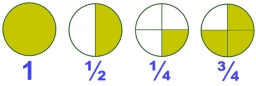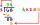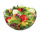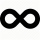# Two divided

Two divided by nine-tenths.

x =  2.2222

### Step-by-step explanation:Did you find an error or inaccuracy? Feel free to write us. Thank you!Tips to related online calculators
Need help to calculate sum, simplify or multiply fractions? Try our fraction calculator.

## Related math problems and questions:

• Double division0.25 divided by 1/2 divided by 14
• Numbers divisionWith what number should be divided mixed number 2 3/4 to get 11/12?
• Evaluate 12Evaluate and simplify 9 divided by 7/6.
• How many 16How many three-tenths are there in two and one-fourths?
• NumberCalculate the integer number which, divided by 34 gives 10 and the rest 25.
• A quotientWhat is the quotient of 3/10 divided by 2/4 as a fraction?
• Equivalent fractions 2Write the equivalent multiplication expression. 2 1/6÷3/4
• JakubJakub multiplied 11 times the number 100 by ten and then divided the result ten times by 10. What number did he get?
• DivisionDivide by the number 0.2 is the same as multiply by what number?
• In dividingIn dividing fractions, get the reciprocal of the divisor and change division symbol to multiplication symbol. 2/3 : 5/6
• Divide 11Divide the product of 4 and 5/8 by 1 1/2. Write your answer as a mixed number.
• Evaluate 11Multiply the quotient of 6 and 2 by 3, then add 1. Add 1 and 6, then divide by 2 and multiply by 3. Divide 6 by the product of 2 and 3, then add 1.We need two tenths kg of a carrot, one tenth of peas and three tenths of of tomatoes to make salad. Express the fraction of the weight of the vegetables to be salad. Convert the result to grams.How many 2/3s are in 6?What is the sign of expression: minus 18 start fraction 9 divided by 17 end fraction plus left parenthesis minus 18 start fraction 9 divided by 17 end fraction right parenthesis?Find the smallest integer: divided by 2, the remainder is 1. divided by 3, the remainder is 2. divided by 4, the remainder is 3. ... divided by eight, the remainder is 7, divided by 9 the remainder is 8.Fraction 5 by 2. if 3 is added to numerator and 2 is subtracted from the denominator then the new fraction is: